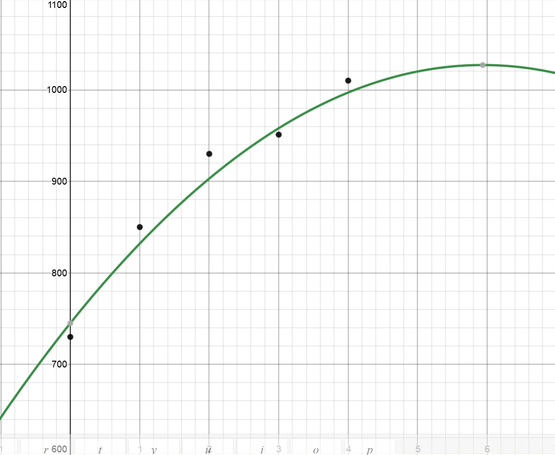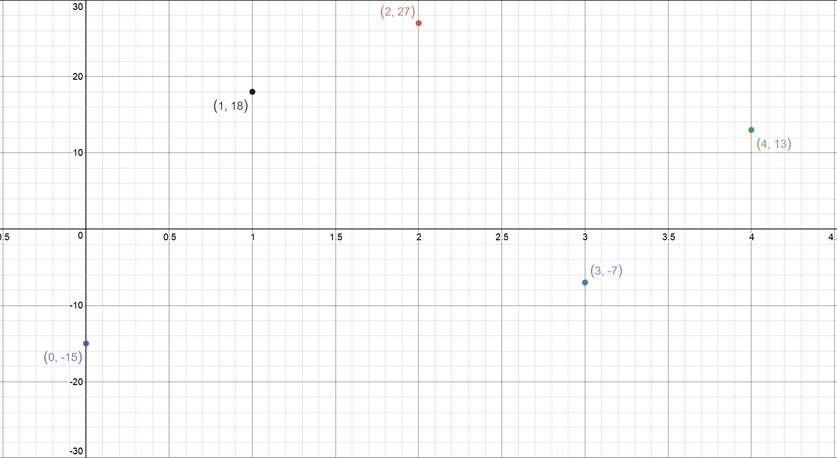# Make a scatterplot of the data and graph the function f(x)= -8x^{2} + 95x + 745.sagnuhh 2021-01-08 Answered

Make a scatterplot of the data and graph the function Make a residual plot and describe how well the function fits the data. $\begin{array}{|cccccc|}\hline \text{Price Increase}& 0& 1& 2& 3& 4\\ \text{Sales}& 730& 850& 930& 951& 1010\\ \hline\end{array}$

You can still ask an expert for help

• Questions are typically answered in as fast as 30 minutes

Solve your problem for the price of one coffee

• Math expert for every subject
• Pay only if we can solve itun4t5o4v

Step 1 By drawing the curve (expected) and marking the points in the graph (actual) we get it is given below. The residual value is calculated as $\begin{array}{|c|}\hline \text{Residual value}=\text{Actual value}-\text{Expected value}\\ \hline\end{array}$

$=\begin{array}{|c|}\hline -15\\ \hline\end{array}$

$=\begin{array}{|c|}\hline +18\\ \hline\end{array}$

$=\begin{array}{|c|}\hline +27\\ \hline\end{array}$

$=\begin{array}{|c|}\hline -7\\ \hline\end{array}$

$=\begin{array}{|c|}\hline +13\\ \hline\end{array}$Step 2 By plotting the residual value to the graph which is given below, we can see that the points are randomly scattered through the graph. This indicates that the line is a Good Fot.